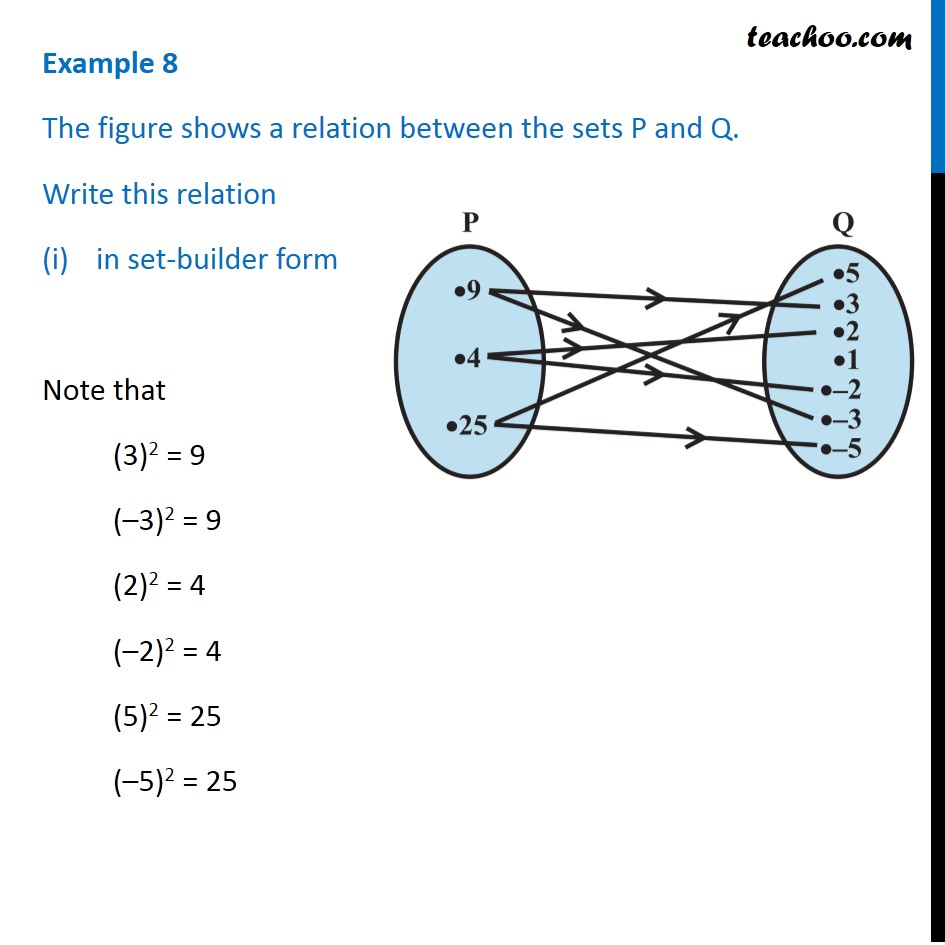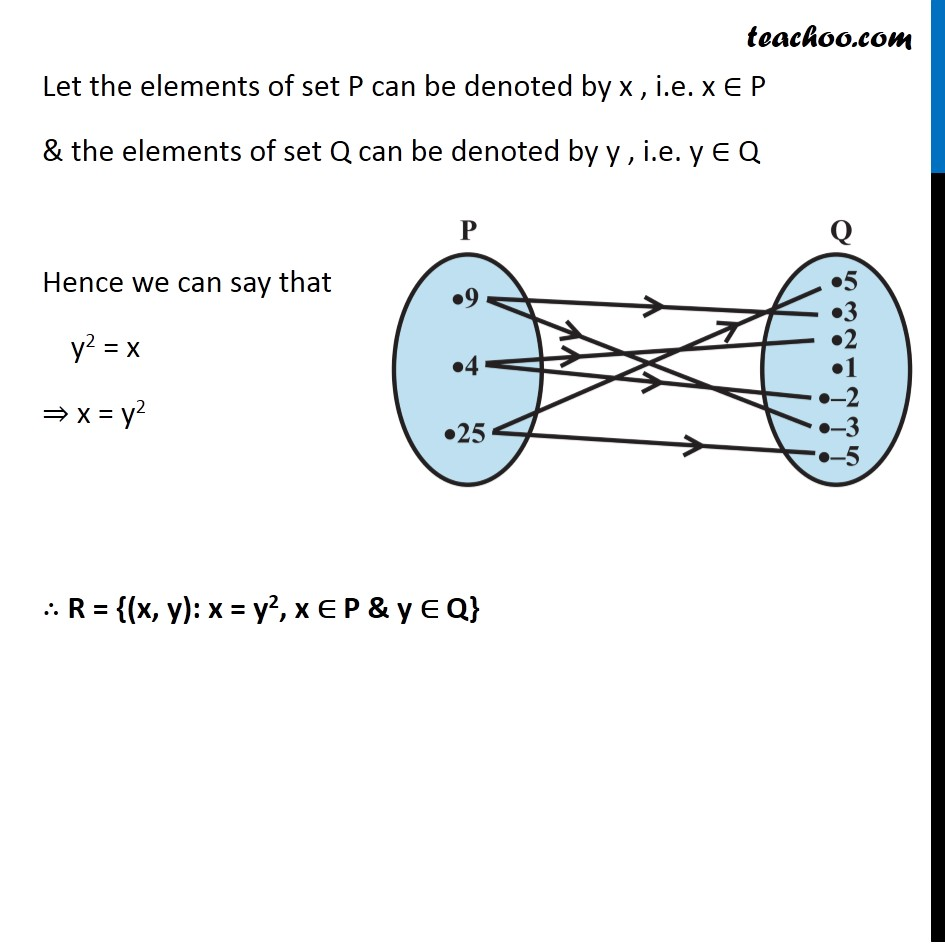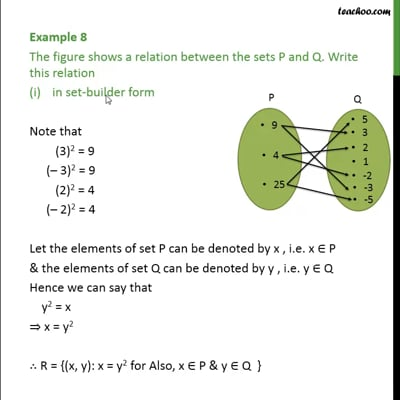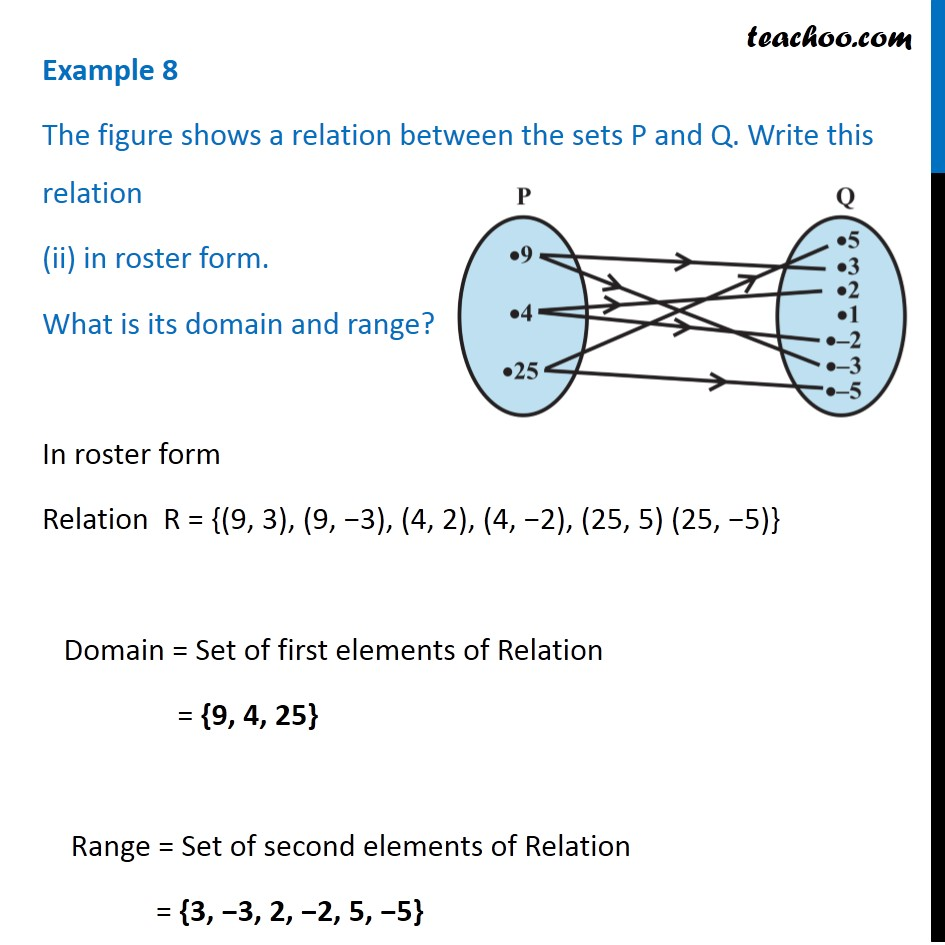Examples

Chapter 2 Class 11 Relations and Functions
Serial order wiseThis video is only available for Teachoo black usersMaths Crash Course - Live lectures + all videos + Real time Doubt solving!

### Transcript

Example 8 The figure shows a relation between the sets P and Q. Write this relation in set-builder form Note that (3)2 = 9 (–3)2 = 9 (2)2 = 4 (–2)2 = 4 (5)2 = 25 (–5)2 = 25 Let the elements of set P can be denoted by x , i.e. x ∈ P & the elements of set Q can be denoted by y , i.e. y ∈ Q Hence we can say that y2 = x ⇒ x = y2 ∴ R = {(x, y): x = y2, x ∈ P & y ∈ Q} Example 8 The figure shows a relation between the sets P and Q. Write this relation (ii) in roster form. What is its domain and range? In roster form Relation R = {(9, 3), (9, −3), (4, 2), (4, −2), (25, 5) (25, −5)} Domain = Set of first elements of Relation = {9, 4, 25} Range = Set of second elements of Relation = {3, −3, 2, −2, 5, −5}